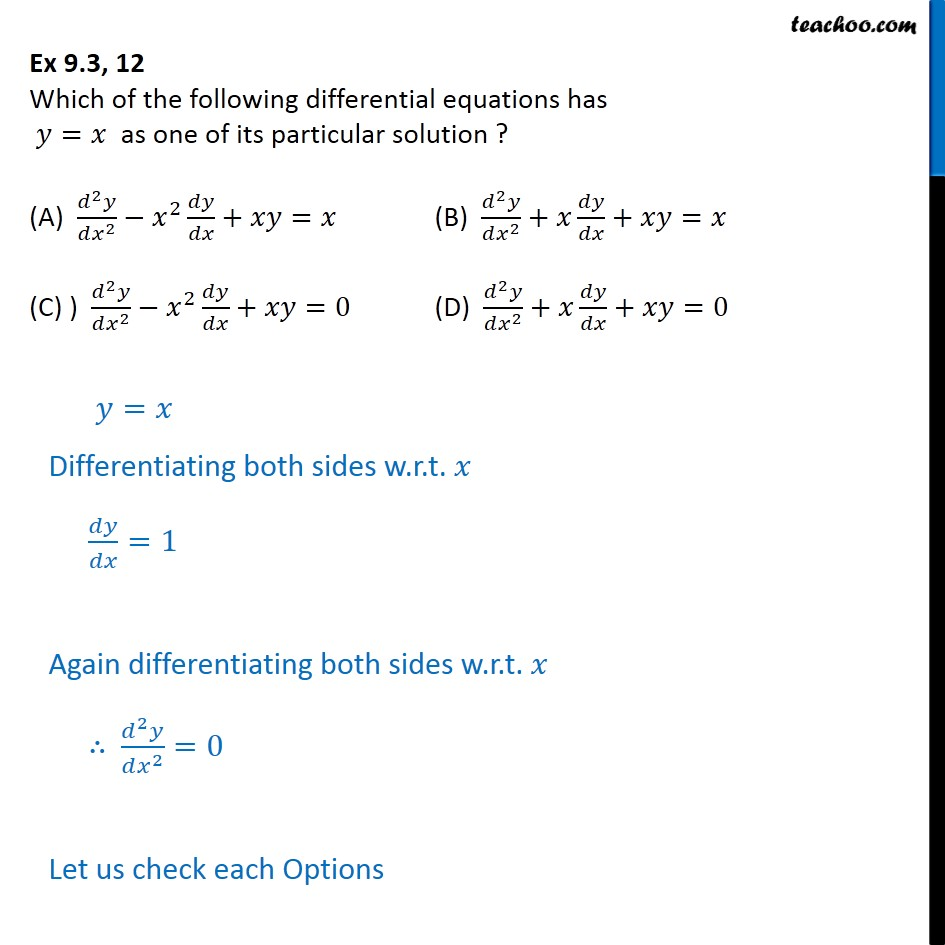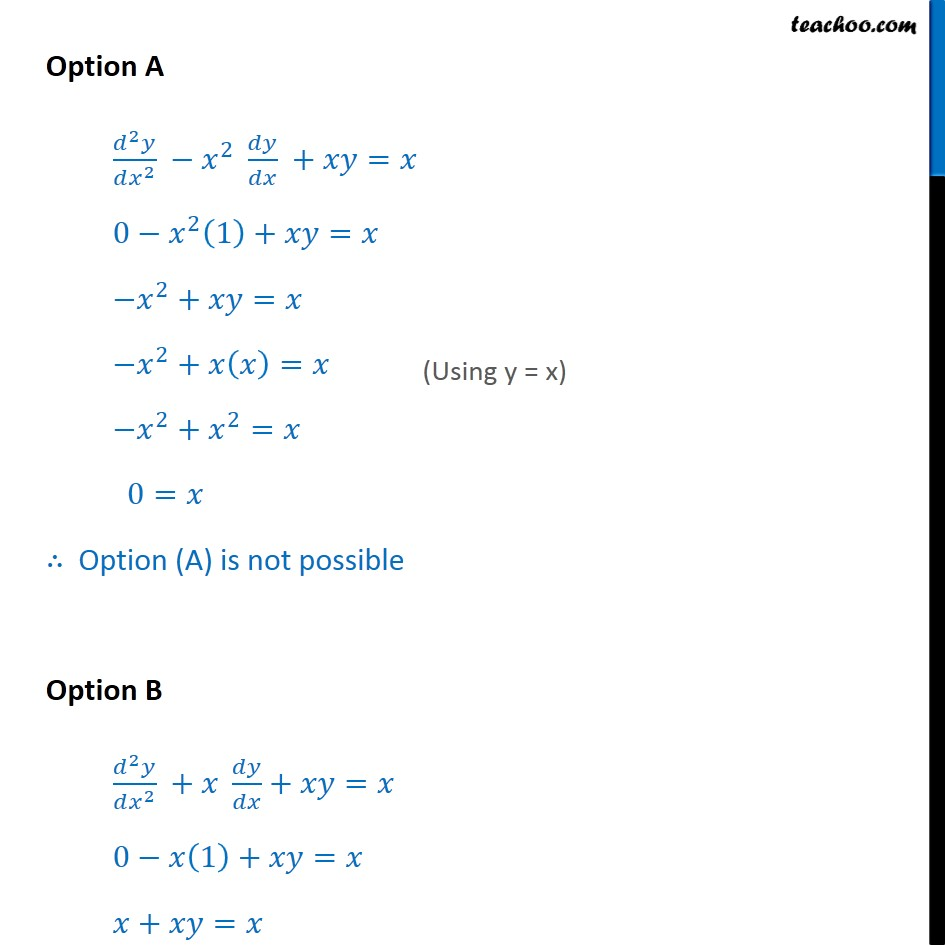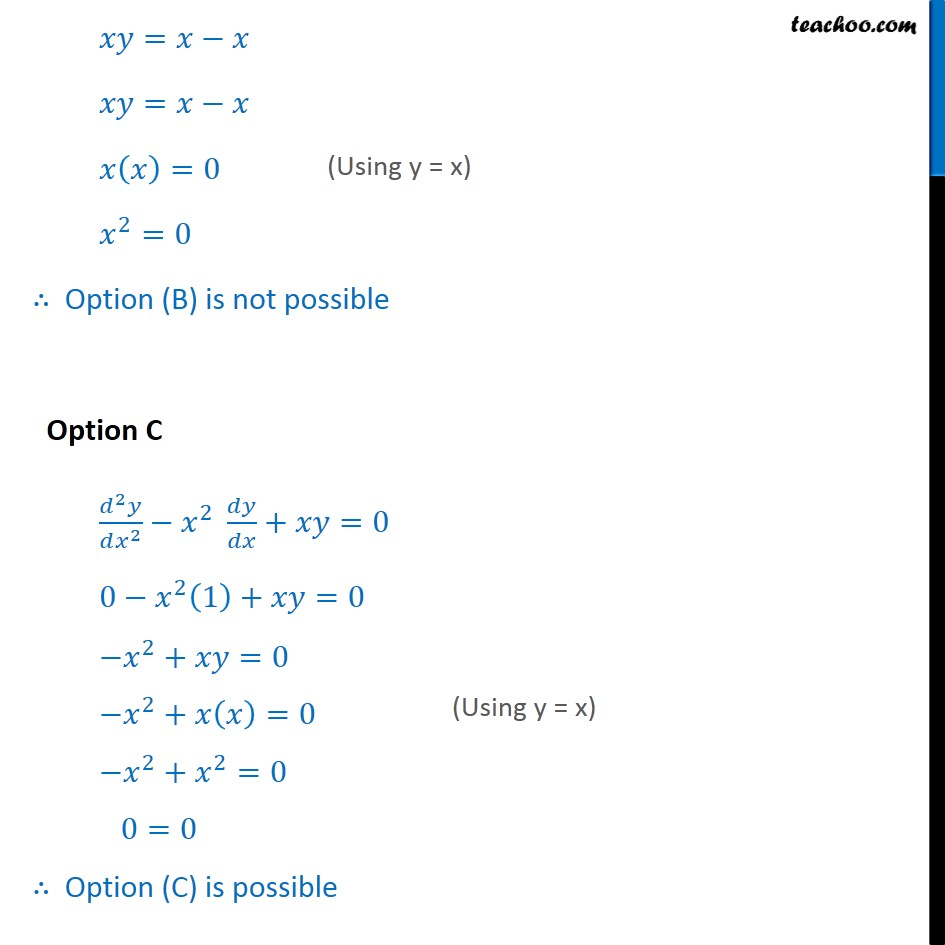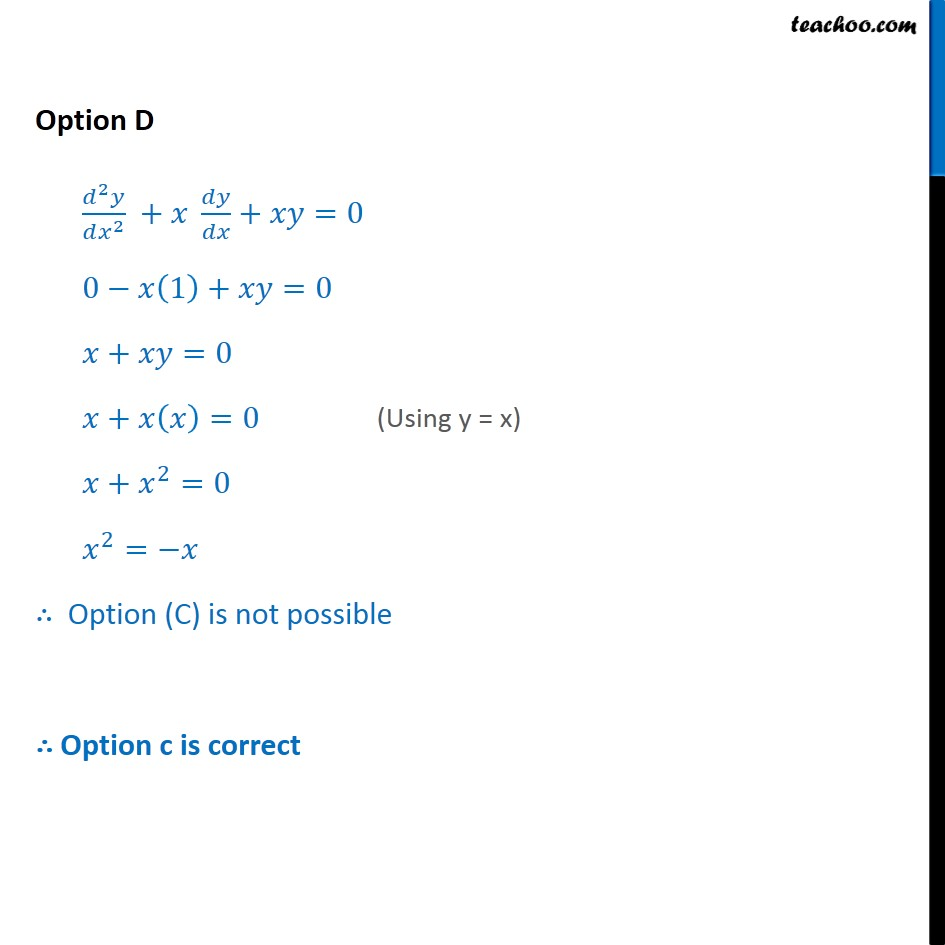1. Chapter 9 Class 12 Differential Equations
2. Serial order wise
3. Ex 9.3

Transcript

Ex 9.3, 12 Which of the following differential equations has 𝑦=𝑥 as one of its particular solution ? (A) (𝑑^2 𝑦)/(𝑑𝑥^2 )−𝑥^2 𝑑𝑦/𝑑𝑥+𝑥𝑦=𝑥 (B) (𝑑^2 𝑦)/(𝑑𝑥^2 )+𝑥 𝑑𝑦/𝑑𝑥+𝑥𝑦=𝑥 (C) ) (𝑑^2 𝑦)/(𝑑𝑥^2 )−𝑥^2 𝑑𝑦/𝑑𝑥+𝑥𝑦=0 (D) (𝑑^2 𝑦)/(𝑑𝑥^2 )+𝑥 𝑑𝑦/𝑑𝑥+𝑥𝑦=0 𝑦=𝑥 Differentiating both sides w.r.t. 𝑥 𝑑𝑦/𝑑𝑥=1 Again differentiating both sides w.r.t. 𝑥 ∴ (𝑑^2 𝑦)/(𝑑𝑥^2 )=0 Let us check each Options Option A (𝑑^2 𝑦)/(𝑑𝑥^2 ) −𝑥^2 𝑑𝑦/𝑑𝑥 +𝑥𝑦=𝑥 0−𝑥^2 (1)+𝑥𝑦=𝑥 −𝑥^2+𝑥𝑦=𝑥 −𝑥^2+𝑥(𝑥)=𝑥 −𝑥^2+𝑥^2=𝑥 0=𝑥 ∴ Option (A) is not possible Option B (𝑑^2 𝑦)/(𝑑𝑥^2 ) +𝑥 𝑑𝑦/𝑑𝑥+𝑥𝑦=𝑥 0−𝑥(1)+𝑥𝑦=𝑥 𝑥+𝑥𝑦=𝑥 𝑥𝑦=𝑥−𝑥 𝑥𝑦=𝑥−𝑥 𝑥(𝑥)=0 𝑥^2=0 ∴ Option (B) is not possible Option C (𝑑^2 𝑦)/(𝑑𝑥^2 )−𝑥^2 𝑑𝑦/𝑑𝑥+𝑥𝑦=0 0−𝑥^2 (1)+𝑥𝑦=0 −𝑥^2+𝑥𝑦=0 −𝑥^2+𝑥(𝑥)=0 −𝑥^2+𝑥^2=0 0=0 ∴ Option (C) is possible Option D (𝑑^2 𝑦)/(𝑑𝑥^2 ) +𝑥 𝑑𝑦/𝑑𝑥+𝑥𝑦=0 0−𝑥(1)+𝑥𝑦=0 𝑥+𝑥𝑦=0 𝑥+𝑥(𝑥)=0 𝑥+𝑥^2=0 𝑥^2=−𝑥 ∴ Option (C) is not possible ∴ Option c is correct

Ex 9.3